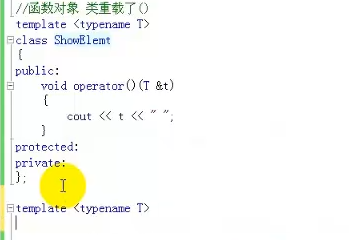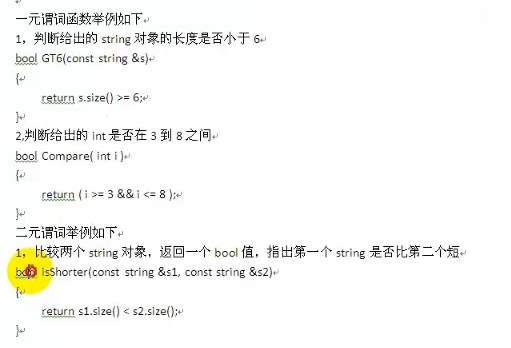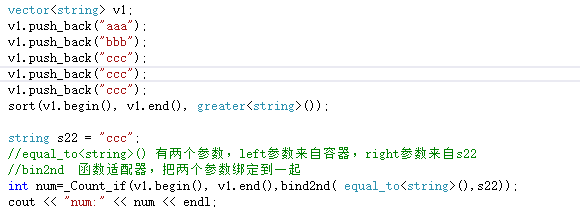c++算法笔记
2018-12-26 19:12:49 qq_23304241 阅读数 111

1、题目描述

``````#include<iostream>
#include<string>
#include<vector>

using namespace std;

int main()
{
string input;
vector<string>arr;
while (cin >> input)
{
arr.push_back(input);
}
cout << arr[arr.size() - 1].length() << endl;
return 0;
}``````

``````#include<iostream>
#include<string>
using namespace std;
int main()
{
string s;
while(getline(cin,s)){
int n=0,flag=1;
for(int i=s.length()-1;i>=0;--i){//倒着计算
if(flag && s[i]==' '){//如果末尾有空格，先清除末尾空格
continue;
}
else if(s[i]!=' '){
flag = 0;
++n;
}
else{
break;
}
}
cout << n << endl;
}
return 0;
}``````

2、题目描述

``````#include<iostream>
#include<string>
#include<ctype.h>
using namespace std;
int main()
{
string str;

while (getline(cin,str))
{
char s;
cin >> s;
int count = 0;
for (int i = 0; i < str.size(); i++)
{
if (tolower(s) == tolower(str[i])) //都转换成小写进行比较
count++;
}
cout << count << endl;
cin.ignore();
}

return 0;
}``````

3、C++中substr函数的用法

``````#include <iostream>
#include <string>
using namespace std;

int main()
{
//string str("123456abc"); string两种初始化方式均可以
string str = "123456abc";
string a = str.substr(6, str.size()); // substr（）可以获得指定字符串
cout << a << endl;   //输出abc
return 0;
}
``````

4、

•连续输入字符串，请按长度为8拆分每个字符串后输出到新的字符串数组；
•长度不是8整数倍的字符串请在后面补数字0，空字符串不处理。

123456789

12345678
90000000

``````#include <iostream>
#include <string>
using namespace std;

void fuck(string str)
{
if (str == "")       //明确分几种情况
return;
if (str.size() <= 8)
{
str.append(8 - str.size(), '0');
cout << str << endl;
return;
}
cout << str.substr(0, 8) << endl;
fuck(str.substr(8, str.size()));//递归调用
}
int main()
{
string str1, str2;
cin >> str1 >> str2;
fuck(str1);
fuck(str2);
return 0;
}
``````

5、合并表并记录

4
0 1
0 2
1 2
3 4

0 3
1 2
3 4

``````#include<iostream>
using namespace std;
int  main()
{
int Key_Value={0},Key,Value,Number,i;
cin>>Number;
for(i=0;i<Number;i++)
{
cin>>Key>>Value;
Key_Value[Key]+=Value;
}
for(i=0;i<10000;i++)
{
if(Key_Value[i]!=0)
cout<<i<<"  "<<Key_Value[i]<<endl;
}
return 0;
}``````

``````#include<iostream>
using namespace std;
struct Key_Value_Pair
{
int index;
int value;
};
int main()
{
Key_Value_Pair Part;
int Key_Value_Pairs_Num,i,j,k;
cin>>Key_Value_Pairs_Num;
for(i=0;i<Key_Value_Pairs_Num;i++)
cin>>Part[i].index>>Part[i].value;
for(i=0;i<Key_Value_Pairs_Num;i++)
{
for(j=i+1;j<Key_Value_Pairs_Num;j++)
{
if(Part[i].index==Part[j].index)
{
Part[i].value+=Part[j].value;//对第二键值求和，累加在第一次出现的键值项上，下一步进行重复索引的删除操作
for(k=j;k<Key_Value_Pairs_Num-1;k++)
Part[k]=Part[k+1];
j--;
Key_Value_Pairs_Num--;
}
}
}
//下面进行排序操作
Key_Value_Pair Temp;//定义一个结构体，作为交换操作的中间值
for(i=0;i<Key_Value_Pairs_Num-1;i++)
{
for(j=0;j<Key_Value_Pairs_Num-1-i;j++)
{
if(Part[j].index>Part[j+1].index)
{
Temp=Part[j];
Part[j]=Part[j+1];
Part[j+1]=Temp;
}
}
}
for(i=0;i<Key_Value_Pairs_Num;i++)
cout<<Part[i].index<<" "<<Part[i].value<<endl;
return 0;
}``````

6、以下代码是把一个字符串倒序，如“abcd”倒序后变为“dcba”。

``````int main()
{
char *src = "hello,world";
int len = strlen(src);
char *dest = (char *)malloc(len + 1); //要为\0分配一个空间
char *d = dest;
char *s = &src[len - 1]; //指向最后一个字符
while ( len-- != 0 )
*d++ = *s--;
*d = 0; //尾部要加\0
printf("%s\n", dest);
free(dest);// 使用完，应当释放空间，以免造成内存汇泄露
return 0;
}``````

``````int main()
{
char str[] = "hello,world";
int len = strlen(str);
char t;
for (int i = 0; i < len; i++)
{
t = str[i];
str[i] = str[len - i - 1];
str[len - i - 1] = t;
}
printf("%s", str);
return 0;
}``````

7、实现函数 ToLowerCase()，该函数接收一个字符串参数 str，并将该字符串中的大写字母转换成小写字母，之后返回新的字符串。

``````class Solution {
public:
string toLowerCase(string str) {
for(auto &c:str){     //对字符串str中的每个字符
c = tolower(c);
}
return str;
}
};``````

8、汉诺塔用递归实现

``````#include <stdio.h>

void hanoi(int n, char A, char B, char C)
{
if(n==1){
printf("%c -> %c\n",A,C);
}
else{
hanoi(n-1,A,C,B);
printf("%c -> %c\n",A,C);
hanoi(n-1,B,A,C);
}

}
int main(){
hanoi(3,'A','B','C');
}``````

9、求数组最大值

``````#include <stdio.h>

int findMax(int arr[], int n){
int max = arr;
int i;
for (i=1;i<n;i++)
{
if (arr[i] > max)
{
max = arr[i];
}
}
return max;
}
int main(){
int arr = {8,2,3,4,6,5,1,10,9,7};
int m= findMax(arr,10);
printf("m = %d\n", m);

return 0;
}

``````

``````#include <stdio.h>

int findMax(int arr[], int L, int R){
if(L == R){
return arr[L];
}
else{
int a = arr[L];
int b = findMax(arr, L+1, R);
if (a > b)
{
return a;
}
else
{
return b;
}
}
}
int main(){
int arr = {8,2,3,4,6,5,1,10,9,7};
int m= findMax(arr,0,9);
printf("m = %d\n", m);

return 0;
}

``````

2018-04-03 21:29:23 Uupton 阅读数 236

二分查找也称折半查找（Binary Search）

1、目的：给定一个数组和一个目标值，返回该目标值在数组中第一次出现位置的下标。

2、限制：（1）该数组必须满足顺序存储结构。（2）必须按关键字大小有序排列。

3、原理：每次取数组正中间的值与目标值比较，若相等则返回该中间值的下标，若目标值大于中间的值则取中间值的后半部分作为一个新的数组(保留原下标)重复第一步的操作，若目标值小于中间的值则取该中间值的前半部分的作为一个新的数组（保留原下标）重复第一步的操作。

```int binarySearch(int num[],int low,int high ,int key)
{
while (low <= high)
{
int mid = (low + high) / 2;
if (num[mid] == key) return mid;
else if (num[mid] > key) high = mid - 1;
else low = mid + 1;
}
return -1;
}```

```int binarySearch(int num[], int low, int high, int key)
{
int mid = (low + high) / 2;
while (low < high)
{
if (num[mid] >= key) high = mid;
else if (num[mid] < key) low = mid + 1;
mid = (low + high) / 2;
}
return num[mid] == key ? mid : -1;
}```

2018-08-03 00:40:17 weixin_37986381 阅读数 66

一、算法概述

1. 算法部分主要头文件<algorithm>,<numeric>,<functional>：<algorithm>是所有STL头文件中最大的一个，常用功能范围有比较、交换、查找、遍历操作、复制、修改、反转、排序、合并；<numeric>体积很小，只包括几个在序列上面进行简单数学运算的模板函数，包括加法和乘法在序列上的一些操作；<functional>中定义了一些模板类，用以声明函数对象。

2. 算法分类：操作对象，直接改变容器的内容，将原容器的内容复制一份，修改其副本，然后传回该附本。非可辨序列算法：指不直接修改其所操作的容器内容的算法（计数算法、搜索算法、比较算法）；可变序列算法：指可以修改他们所操作的内容的算法（删除算法、修改算法、排序算法）

3. 算法中的函数对象和谓词：

4. 函数对象--》重载函数调用操作符的类，其对象常称为函数对象，即他们是行为类似函数的对象，一个类对象，表现出一个函数的特征，就是通过“对象名+（参数列表）”方式使用一个类对象，如果没有上下文，完全可以把它看作一个函数对待。这是通过重载类的operator()来实现的。“在标准库中的很对算法都可以使用函数对象或者函数来作为自定回调行为。---实现了数据类型和算法的分离1. 谓词--》一元函数对象，函数参数1个；二元函数参数对象，函数参数2个。一元谓词：函数参数一个，函数返回值是bool类型，可以作为一个判断式；谓词可以是一个仿函数，也可以是一个回调函数；二元位置，函数参数2个，函数返回值是bool类型。

2.5.函数适配器二、STL常用的算法

1. for_each()
2. for_each()：用指定函数一次对指定范围内所有元素进行迭代访问，该函数不得修改序列中的元素。for_each()的第三个参数，函数对象做函数参数，函数对象做返回值。
``````#include <iostream>

using namespace std;

#include "string"
#include "set"
#include <vector>
#include <list>
#include <algorithm>
#include "functional"

void printV(vector<int> &v)
{
for (vector<int>::iterator it = v.begin(); it != v.end(); it++)
{
cout << *it << "\t";
}
}
void showElem(int &n)
{
cout << n << "\t";
}
class CMyShow
{

public :
CMyShow()
{
num = 0;
}
void operator()(int &n)
{
cout << n << "\t";
num++;
}
void printV()
{
cout << num << endl;
}
private :
int num;
};
void main41()
{
vector<int> v1;
v1.push_back(1);
v1.push_back(3);
v1.push_back(5);
printV(v1);
cout << endl;
for_each(v1.begin(), v1.end(), showElem);
cout << endl;
CMyShow mya;
CMyShow my1=for_each(v1.begin(), v1.end(), mya);
cout << endl;
my1.printV();//my1和mya是两个不同的对象
mya.printV();
}

void main()
{
main41();
cout << endl;
system("pause");
}``````

3. transform():与for_each类似，遍历所有元素，但可对容器的元素进行修改。他可以把一个容器的呀U尿素，通过op，变换到另一个容器中（同一个容器中）；也可以把两个容器的元素，通过op，变换到另一个容器中。
``````#include <iostream>

using namespace std;

#include "string"
#include "set"
#include <vector>
#include <list>
#include <algorithm>
#include "functional"
#include "iterator"
void printV(vector<int> &v)
{
for (vector<int>::iterator it = v.begin(); it != v.end(); it++)
{
cout << *it << "\t";
}
}
void printList(list<int> &v)
{
for (list<int>::iterator it = v.begin(); it != v.end(); it++)
{
cout << *it << "\t";
}
}

int increase(int i)
{
return i + 100;
}

void main42()
{
vector<int> v1;
v1.push_back(1);
v1.push_back(3);
v1.push_back(5);
printV(v1);
cout << endl;
//使用回调函数
transform(v1.begin(), v1.end(), v1.begin(), increase);
printV(v1);
cout << endl;

//使用预定义的函数对象
transform(v1.begin(), v1.end(), v1.begin(), negate<int>());
printV(v1);
cout << endl;

//使用函数适配器
list<int> mylist;
mylist.resize(v1.size());
transform(v1.begin(), v1.end(), mylist.begin(),bind2nd(multiplies<int>(),10));
printList(mylist);
cout << endl;

//把运算结果直接输出到屏幕
transform(v1.begin(), v1.end(), ostream_iterator<int>(cout, "\t"), negate<int>());

}
void main()
{
main42();
cout << endl;
system("pause");
}``````

4.   for_each与transform的区别   一般情况下，for_each所使用的函数对象，参数是引用，没有返回值；tranform所使用的函数对象，参数一般不使用引用，而且还有返回值；
5.
6.
2019-03-03 09:36:02 qq_23304241 阅读数 36

1、输入一个链表，输出该链表中倒数第k个结点

``````class Solution{
public:
ListNode * FindKthToTail(ListNode * pListHead, unsigned int k)
{
int i = 0;
for (; p != NULL; i++)
{
if (i >= k)
{
q = q->next;
}
p = p->next;
}
return i > k ? NULL : q;
}
};``````

2、判断字符串是否为回文

``````#include <iostream>
#include <string>
using namespace std;
int main()
{
int i, j,l;
string str;
int flag = 1;
while (cin >> str)
{
l = str.length();

for (i = 0, j = l - 1; i <= j; i++, j--)
{
if (str[i] != str[j])
{
flag = 0;
break;
}
}
if (flag)
{
cout<<"YES"<< endl;
}
else
{
cout << "NO" << endl;
}
flag = true;
}
return 0;
}``````

2017-12-07 20:15:38 weifenglin1997 阅读数 157

y=a1*p(n-1)+a2*p(n-2)+....+an-1p+an

``` int x,p;
int y=0,pro=1;//p表示进制
while(x!=0){
y=y+(x%10)*pro;
x=x/10;
pro=p*pro;
}```
//将 十进制转换为q进制，除基取余法；
/*例如11转换为2进制

5%2=2  余1
2%2=1  余0
1%2=0  余1

*/
int z,num=0;
do{
z[num++]=y%q;
y=y/q;

}while(y!=0);//当商不为0时循环

100 ms

65536 kB

8000 B

Standard

CHEN, Yue

```123 456 8
```

`1103`

```#include <stdio.h> #include<iostream> #include <string.h> #include<stdlib.h> using namespace std; //进制转换 int main(){ int a,b,y,q; scanf("%d%d%d",&a,&b,&q); y=a+b; int z,num=0; do{ z[num++]=y%q; y=y/q; }while(y!=0);//当商不为0时循环 for(int i=num-1;i>=0;i--){ cout<<z[i]; } return 0; } ```

c++算法刷题笔记

c++算法刷题笔记字符串处理字符串处理1.对于从键盘接受HelloWorld这样存在空格的输入，不可以使用cin，可以使用形如stringch;getline(cin,ch);来接受一行字符串2.将string类转换成char[]时，可以使用strcpy(ch,str.c_str());，需要包含头文件#include&amp;amp;amp;amp;lt;string.h&amp;amp;amp;amp;gt;...